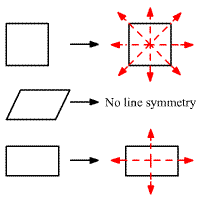# Line Symmetry

A figure has symmetry if there is a transformation of the figure such that the image coincides with the preimage.

The line of symmetry (line symmetry or reflection symmetry) of a figure is a line that divides the figure into two congruent parts which are mirror image of each other.

Example:

A square has $4$ lines of symmetry, a parallelogram has no lines of symmetry, and a rectangle has $2$ lines of symmetry.